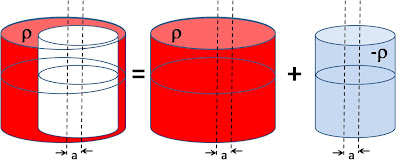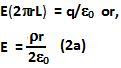## Friday, May 7, 2010

### Irodov Problem 3.29The solution to this problem exactly the same as that in Problem 3.28, except that we now have a cylinder instead of a sphere - i.e. using superposition principle. The cylinder with the cavity is same as the superposition of a solid cylinder with charge densityand a smaller cylindercorresponding to the cavity of density.

First let us compute the electric field at a distance r from the axis inside a solid uniformly charged cylinder. Similar to in problem 3.28 we consider a cylindrical Gaussian surface of radius r and a very large length L about the axis of the cylinder. The total charge in the cylinder will be,Then from Gauss's Law we have,If we consideras a planar vector in the horizontal plane from the axis as shown in figure 2, then,Letbe the planar vector drawn from the axis of the cylinder to the axis of the cavity, then as in Problem 3.28, the net electric field is given by,#### 1 comment:

1.Electric Field Intensity
It is the force experienced by a unit positive charge placed at a point in an electric field.

Due to a point charge

Due to linear distribution of charge

λ = linear charge density of rod

(i) At a point on its axis.

(ii) At a point on the line perpendicular to one end

Due to ring of uniform charge distribution
At a point on its axis

Due to uniformly charged disc.
At a point on its axis.

Thin spherical shell

1. Non conducting solid sphere having uniform volume distribution of charge
(i) Outside point

(ii) Inside point
q = total charge
ρ = volume density of charge

Cylindrical Conductor of Infinite length

Outside point

Inside point λ = linear density of charge
E = 0 (as charges reside only on the surface)
Non-conducting cylinder having uniform volume density of charge

Infinite plane sheet of charge

λ = surface charge density
Two oppositely charged sheets (Infinite)
(i) Electric field at points outside the charged sheets
EP = ER = 0
(ii) Electric field at point in between the charged sheets

Example 3. Find electric field intensity due to long uniformly charged wire.
(charge per unit length is λ)